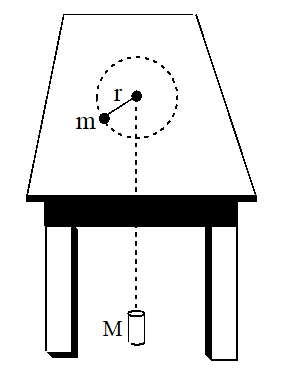A puck is moving in a circle of radius r with a constant speed  V0 on a level frictionless table. A string is attached to the puck which holds it in the circle. The string passes through a frictionless hole and is attached on the other end to a hanging object of mass M. The puck is now made to move with a speed v=3v0 but still in circle. The mass of the hanging object is left unchanged. The acceleration a of the puck and the radius r of the circle are now given by (a0 is the initial acceleration of the puck)

# A puck is moving in a circle of radius r with a constant speed  ${V}_{0}$ on a level frictionless table. A string is attached to the puck which holds it in the circle. The string passes through a frictionless hole and is attached on the other end to a hanging object of mass M. The puck is now made to move with a speed $v=3{v}_{0}$ but still in circle. The mass of the hanging object is left unchanged. The acceleration a of the puck and the radius r of the circle are now given by (a0 is the initial acceleration of the puck)1. A

${a}^{\text{'}}=9{a}_{0}\text{\hspace{0.17em}}and\text{\hspace{0.17em}}{r}^{\text{'}}={r}_{0}$

2. B

${a}^{\text{'}}={a}_{0}\text{\hspace{0.17em}}and\text{\hspace{0.17em}}{r}^{\text{'}}=9{r}_{0}$

3. C

${a}^{\text{'}}=9{a}_{0}\text{\hspace{0.17em}}and\text{\hspace{0.17em}}{r}^{\text{'}}=9{r}_{0}$

4. D

${a}^{\text{'}}={a}_{0}\text{\hspace{0.17em}}and\text{\hspace{0.17em}}{r}^{\text{'}}={r}_{0}$

Register to Get Free Mock Test and Study Material

+91

Verify OTP Code (required)

### Solution:

$T=\frac{m{V}^{2}}{R},and\text{\hspace{0.17em}}T=mg$

$\begin{array}{l}\frac{m{v}_{0}^{2}}{{r}_{0}}=mg\left(v={v}_{0},r={r}_{0}\right)\\ \frac{m{\left(3{v}_{0}\right)}^{2}}{r\text{'}}=mg\\ r\text{'}=9{r}_{0}\end{array}$
${a}_{0}=\frac{{v}_{0}^{2}}{{r}_{0}}$
$a\text{'}=\frac{{\left(3{v}_{0}\right)}^{2}}{9{r}_{0}}$
$=a\text{'}={a}_{0}$Register to Get Free Mock Test and Study Material

+91

Verify OTP Code (required)